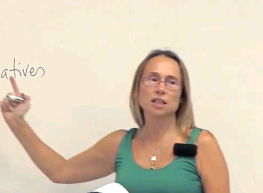# Math 2B: Calculus (English)

## Course Information

Math 2B is the second quarter of Single-Variable Calculus and covers the following topics: Definite integrals; the fundamental theorem of calculus. Applications of integration including finding areas and volumes. Techniques of integration. Infinite sequences and series. Parametric and polar equations.

Mathematics Dept. | Physical Sciences Sch. | University of California, Irvine
Keywords: mathematics,calculus,definite integrals,indefinite integrals,substitution rule,integration strategies,L'Hospital Rule,sequences and series,Power series,Taylor series,comparison test,Maclaurin seriesAuthor:
Natalia Komarova
Title:
Professor
Department:
Mathematics